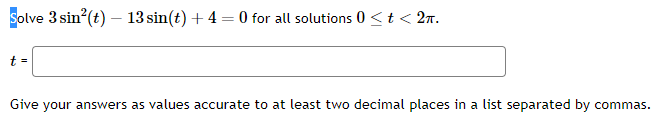# (Solved): Solve 3sin2(t)13sin(t)+4=0 for all solutions 0t<2 Give your answers as values accurate ...Solve for all solutions Give your answers as values accurate to at least two decimal places in a list separated by commas.

We have an Answer from Expert

given trigonometric equation:
where

Factor the left side of equation:

We have an Answer from Expert